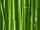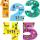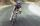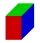# Expression of a variable from the formula - 9th grade (14y) - math problems

#### Number of problems found: 554

• BambooBamboo high 32 feet was at a certain height broken by the wind so the bamboo top reached the ground at a distance of 16 feet from the trunk. At what height from the ground was the bamboo broken?
• Circle's chordsIn the circle there are two chord length 30 and 34 cm. The shorter one is from the center twice than longer chord. Determine the radius of the circle.
• Trapezoid - central medianThe central median divides the trapezoid into two smaller trapezoids. Determines the ratio of their contents.
• CoefficientDetermine the coefficient of this sequence: 7.2; 2.4; 0.8
• The volumeThe volume of the sphere is 1 m3, what is its surface?
• Unknown numberDetermine the unknown number, which double of its fourth square is equal the fifth its square.
• TetrahedronCalculate height and volume of a regular tetrahedron whose edge has a length 4 cm.
• ChordIn a circle with radius r=60 cm is chord 4× longer than its distance from the center. What is the length of the chord?
• TV towerCalculate the height of the television tower if an observer standing 430 m from the base of the tower sees the peak at an altitude angle of 23°?
• Two cyclistsTwo cyclists started from crossing in the same time. One goes to the north speed 20 km/h, the second eastward at speed 26 km/h. What will be the direct distance cycling 30 minutes from the start?
• Sphere cutA sphere segment is cut off from a sphere k with radius r = 1. The volume of the sphere inscribed in this segment is equal to 1/6 of the volume of the segment. What is the distance of the cutting plane from the center of the sphere?
• Equilateral triangleCalculate the area of an equilateral triangle with circumference 72cm.
• Equilateral triangleThe equilateral triangle has a 23 cm long side. Calculate its content area.Determine the radius of the circle, if its perimeter and area is the same number.
• In theIn the rectangle ABCD, the distance of its center from the line AB is 3 cm greater than from the line BC. The circumference of the rectangle is 52 cm. Calculate the contents of the rectangle. Express the result in cm2.
• Car and cyclistCyclist started from town at speed 18 km/h. After 1 hour 30 min started the car from town and caught up with the cyclist in 50 minutes. How fast was a car?The ladder touch on a wall at a height of 7.5 m. The angle of the inclination of the ladder is 76°. How far is the lower end of the ladder from the wall?
• Broken treeThe tree was 35 meters high. The tree broke at the height of 10 m above the ground. Top but does not fall off it refuted on the ground. How far from the base of the tree lay its peak?
• Find theFind the surface of the cuboid if its edges have the dimensions a, 2/3a, 2a
• The volume 2The volume of a cube is 27 cubic meters. Find the height of the cube.

Do you have an interesting mathematical word problem that you can't solve it? Submit a math problem, and we can try to solve it.

We will send a solution to your e-mail address. Solved examples are also published here. Please enter the e-mail correctly and check whether you don't have a full mailbox.

Please do not submit problems from current active competitions such as Mathematical Olympiad, correspondence seminars etc...